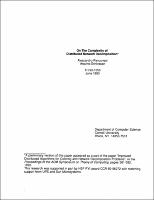## On the Complexity of Distributed Network Decomposition##### Abstract
In this paper, we improve the bounds for computing a network decomposition, which is a basic notion in distributed graph algorithms, distributively and deterministically. Our algorithm computes an $(n^{\epsilon(n)},(n^{\epsilon(n)})$-decomposition in $O(n^{\epsilon(n)})$ time, where $\epsilon(n)=O(1/ \sqrt{\log n})$. As a corollary we obtain improved deterministic bounds for distributively computing several graph structures such as maximal independent sets and $\Delta$-vertex colorings. We also show that the class of graphs $\cal G$ whose maximum degree is $O(n^{\delta(n)})$, where $\delta(n)=O(1/\log \log n)$, is complete for the task of computing a near-optimal decomposition, i.e., a $(\log n \log n)$-decomposition, in $O($polylog$(n))$ time. This is a corollary of a more general characterization, which pinpoints the weak points of existing network decomposition algorithms. Completeness is to be intended in the following sense: if we have an algorithm $\cal A$ that computes an optimal decomposition in $O($polylog$(n))$ time for graphs in $\cal G$, then we can compute an optimal decomposition in $O($polylog $(n))$ time for all graphs.
1993-06
##### Publisher
Cornell University
##### Keywords
computer science; technical report
##### Previously Published As
http://techreports.library.cornell.edu:8081/Dienst/UI/1.0/Display/cul.cs/TR93-1358
technical report